Examples

Chapter 12 Class 10 Surface Areas and Volumes
Serial order wise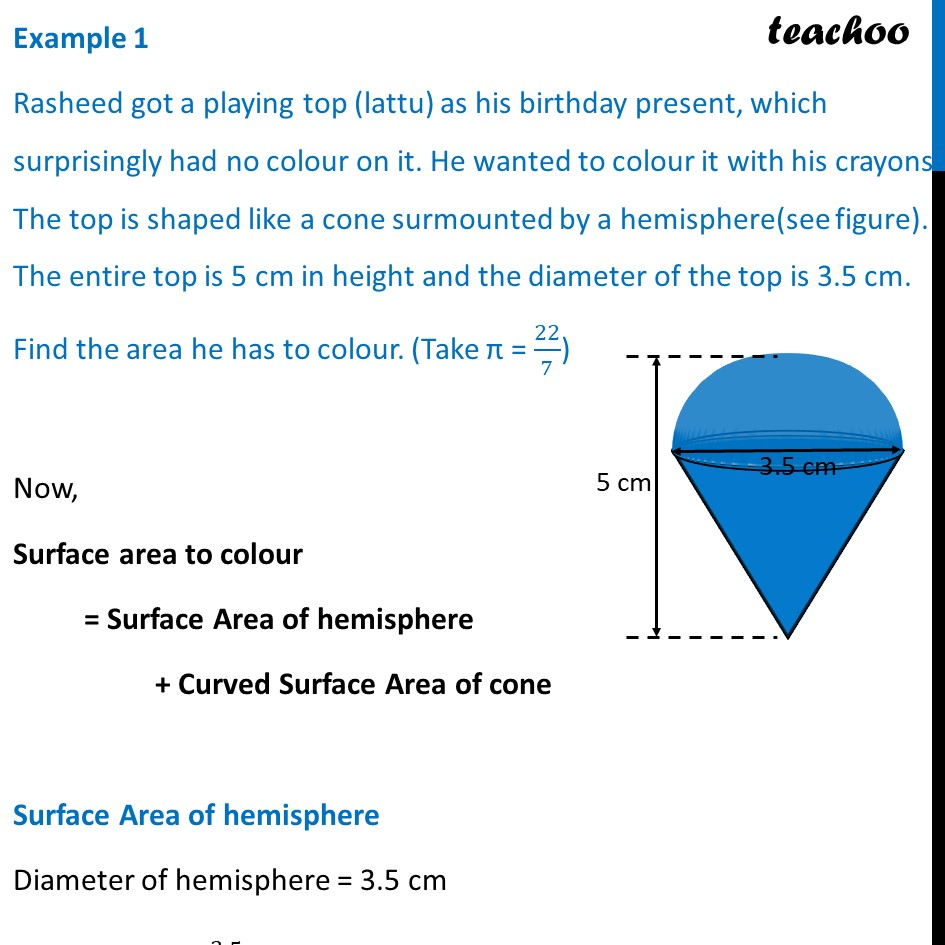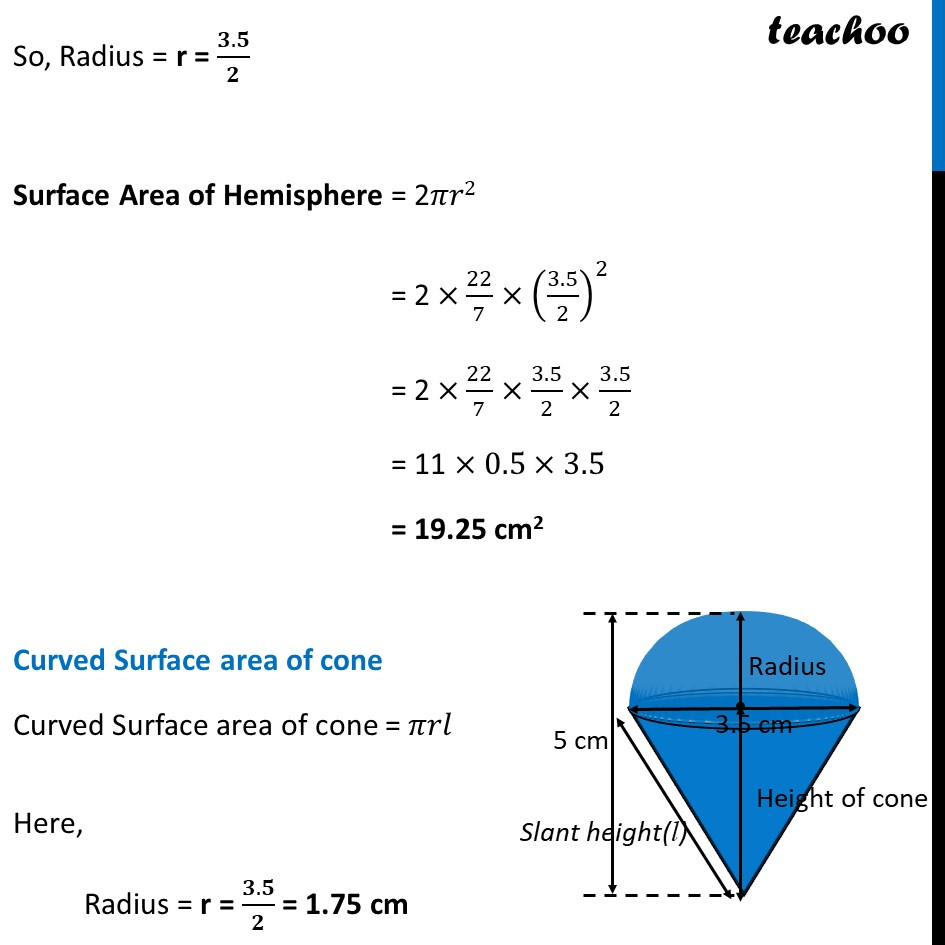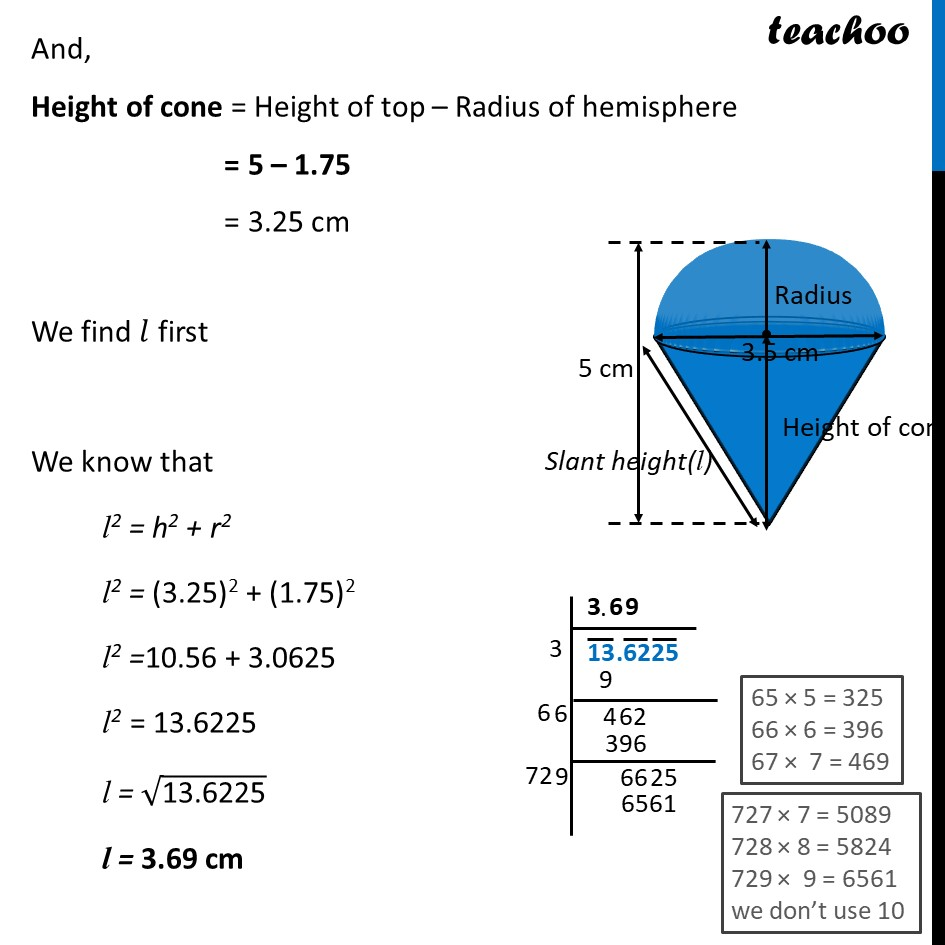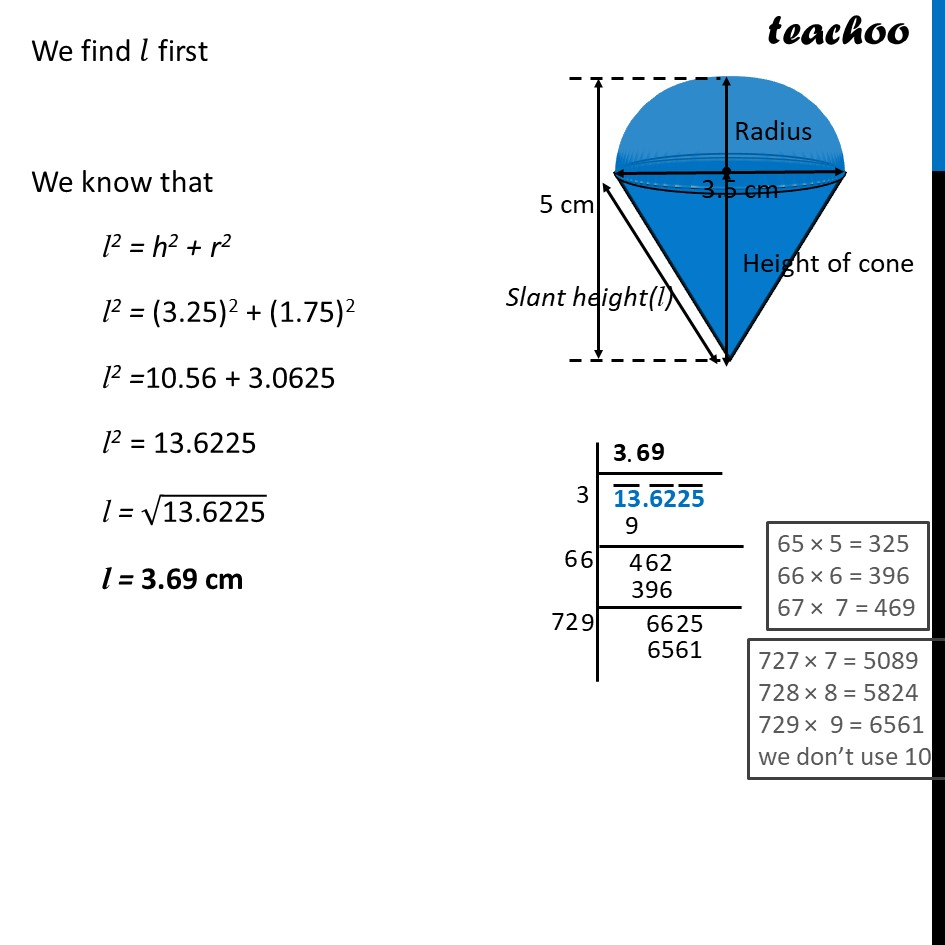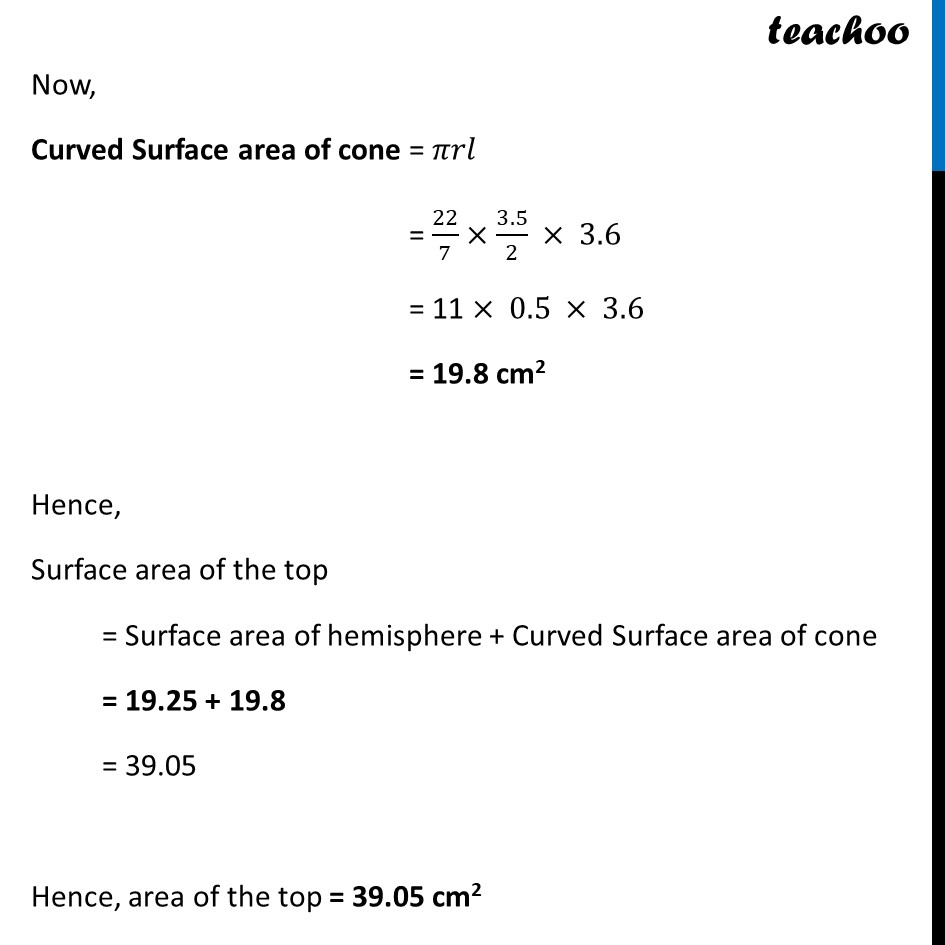Learn in your speed, with individual attention - Teachoo Maths 1-on-1 Class

### Transcript

Example 1 Rasheed got a playing top (lattu) as his birthday present, which surprisingly had no colour on it. He wanted to colour it with his crayons. The top is shaped like a cone surmounted by a hemisphere(see figure). The entire top is 5 cm in height and the diameter of the top is 3.5 cm. Find the area he has to colour. (Take π = 22/7) Now, Surface area to colour = Surface Area of hemisphere + Curved Surface Area of cone Surface Area of hemisphere Diameter of hemisphere = 3.5 cm So, radius = r = 3.5/2 Surface Area of hemisphere = 2𝜋𝑟2 = 2 ×22/7×(3.5/2)^2 So, Radius = r = (𝟑.𝟓)/𝟐 Surface Area of Hemisphere = 2𝜋𝑟2 = 2 ×22/7×(3.5/2)^2 = 2 ×22/7×3.5/2×3.5/2 = 11 ×0.5×3.5 = 19.25 cm2 Curved Surface area of cone Curved Surface area of cone = 𝜋𝑟𝑙 Here, Radius = r = (𝟑.𝟓)/𝟐 = 1.75 cm And, Height of cone = Height of top – Radius of hemisphere = 5 – 1.75 = 3.25 cm We find 𝑙 first We know that l2 = h2 + r2 l2 = (3.25)2 + (1.75)2 l2 =10.56 + 3.0625 l2 = 13.6225 l = √("13.6225" ) l = 3.69 cm We find 𝑙 first We know that l2 = h2 + r2 l2 = (3.25)2 + (1.75)2 l2 =10.56 + 3.0625 l2 = 13.6225 l = √("13.6225" ) l = 3.69 cm Now, Curved Surface area of cone = 𝜋𝑟𝑙 = 22/7×3.5/2 × 3.6 = 11 × 0.5 × 3.6 = 19.8 cm2 Hence, Surface area of the top = Surface area of hemisphere + Curved Surface area of cone = 19.25 + 19.8 = 39.05 Hence, area of the top = 39.05 cm2